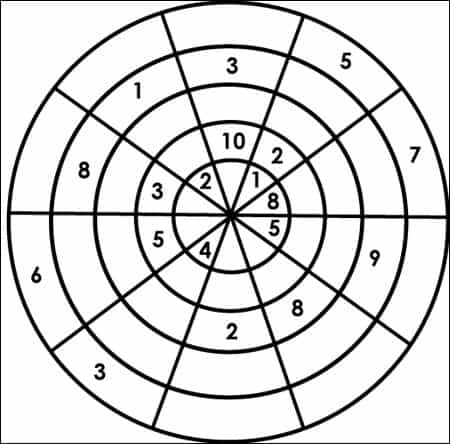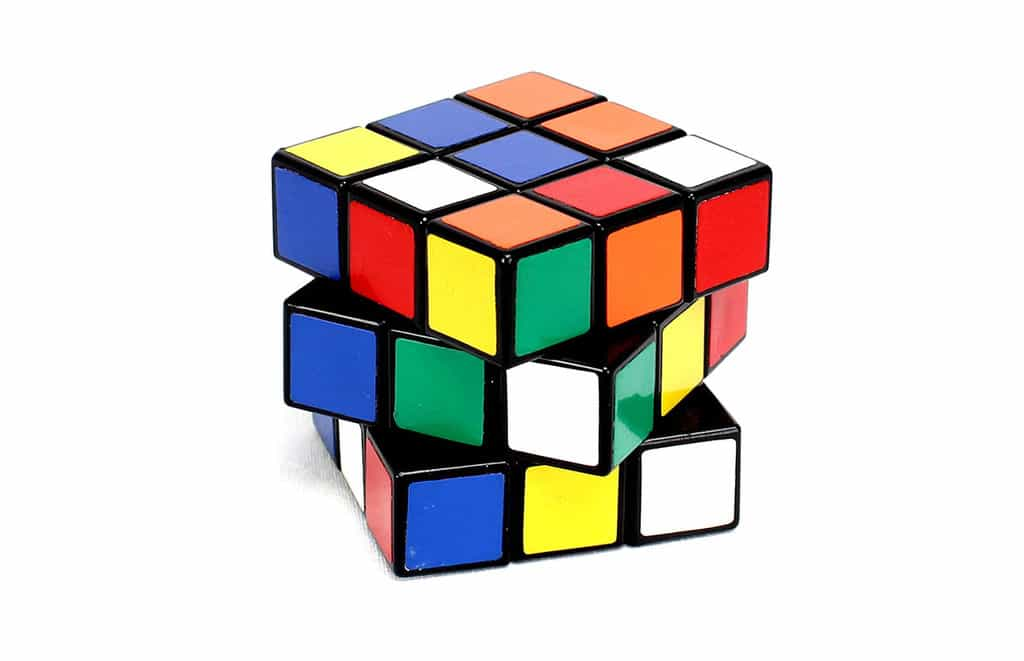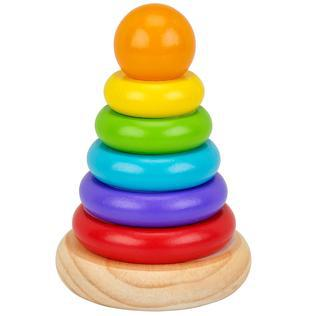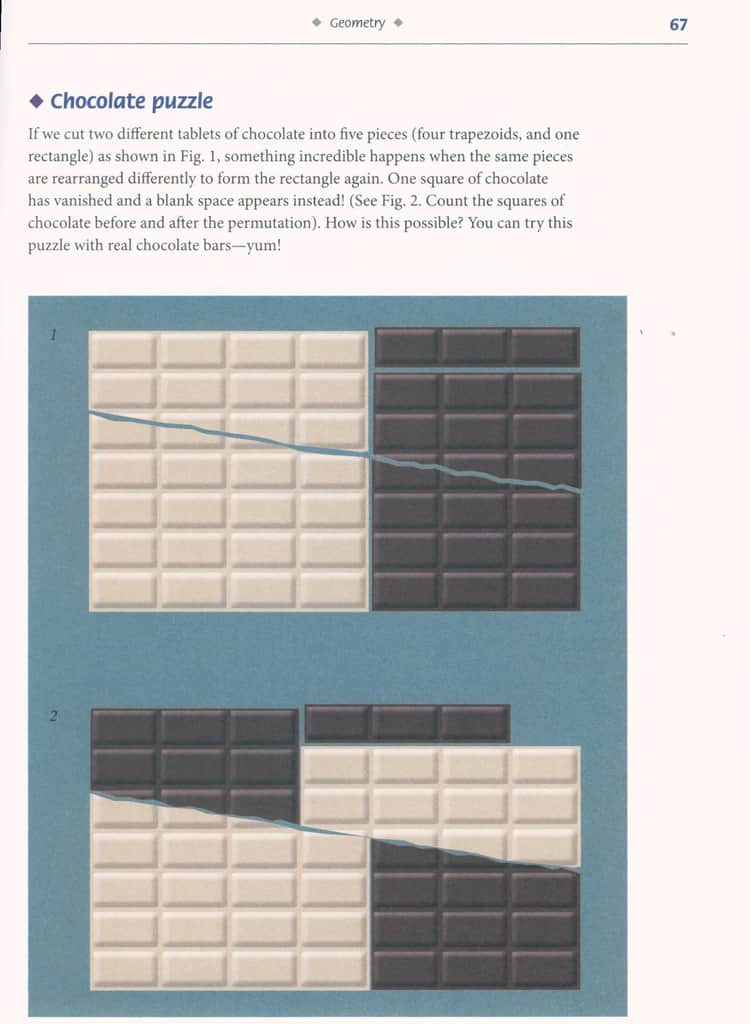## It’s National Puzzle Day!!

Are you addicted to Sudoku? Rubik’s Cube© ? Logic puzzles?

Well, you are in luck, because —

### January 29th is National Puzzle Day!!

• Nothing is a difficult as it seems
• Nothing is as easy as it looks
• Puzzles always have one, several, or no solutions
(Gianni A. Sarcone in the introduction to Impossible Folding Puzzles and Other Mathematical Paradoxes)

SO many puzzles! Where does one even begin!?

How much time did you spend playing tick-tac-toe when you were a kid?  Did you realize the person who had the first go was at a disadvantage? The first player actually has to draw one connecting line longer than the opponent.  So, if you are the first to go and still win, that’s impressive! If you add more squares – say 18 – there are 153 connecting lines. Which means there are 3153 game situations – roughly equivalent to the number of particles in the universe. Searching for a winning strategy is quite impossible and sometimes referred to as “computational chaos.” I had trouble winning with just 9 squares….

Another popular grid puzzle is Sudoku. The most common version of the puzzle consists of 9 squares by 9 squares – a grid of 81 squares. The grid is divided into 9 blocks, each containing 9 squares. The rules: each of the 9 blocks must contain all the numbers 1 – 9 within the squares. Each number can only appear once in a row, column or box. The tricky part is that each vertical 9-square column or horizontal 9-square line – within the larger square – must also contain each of the numbers 1 – 9, with no repeats… Each puzzle has only one solution…If that isn’t challenging enough, there are also circular Sudoku puzzles!

Each of the 4 rings and 8 quarter circles have the numbers 1 through 8 (unlike the square version which has 9). Of course, you can always have 3-ring puzzles, or 5 and 6 ring puzzles. Variants and puzzles can be found in Nets, Puzzles, and Postmen.

How many of us have tried to solve the Rubik’s Cube© ?The classic Rubik’s Cube© consists  of 26 cubelets on 3 levels. Each level of cubelets can be twisted by 90 or 180 degrees. If you twist the layers independently the cube can be brought into approximately 43 million, trillion possible states of the cube (yes, really 43 million trillion!) … The goal? Make each side of nine cubelets the same color. Tomas Rokicki, a Stanford trained mathematician, ran a program on the supercomputer at Sony Pictures Imageworks. The computing time required the equivalent of 50 years of computing – and with solving more than 25 million billion configurations none were recorded that required fewer than 22 moves. Are you able to do it in 22 moves? Are you able to do it in fewer than 22 moves?

Are you able to solve it in a minute and a half? This robot made with Legos© and Raspberry Pi (we have the Raspberry Pi 2 in our Tool Library!) can!Ready for the grown-up version of the baby donut stacker?

The Tower of Hanoi is a much more complicated form of the donut stacker. It was invented by the French mathematician, Edouard Lucas, and was first sold as a toy in 1883. The goal is to transfer the tower of 8 disks to one of the 2 vacant pegs in the fewest moves possible…without putting a larger disk on a smaller one…. For 8 disks that will take 255 moves… If you haven’t figured it out for yourself, the complete mathematical formulas are on pages 196-200 in Famous Puzzles of Great Mathematicians.Logic puzzles more your style? Try to solve these – good luck!!

• Wine & Water:
• A dishonest servant takes 3 pints of wine from a barrel and replaces those pints with the same amount of water. He repeats his theft twice, removing a total of 9 pints, replacing those pints with water. As a result, the diluted wine remaining in the barrel lost half of its former strength. How much wine did the barrel originally hold?
• Animals in a field:
• A cow, a goat, and a goose graze on grass in a field. The cow eats the same quantity of grass as the goat and the goose together. the cow and the goat eat all of the grass in the field in 45 days, the cow and the goose in 60 days, and the goat and the goose in 90 days. How many days will it take the cow, the goat, and the goose together to eat all of the grass, assuming that the grass grows at the same daily rate?
• Compose plane figures/Fibonacci’s numbers:
• Make a rectangle without any gaps by using small squares whoe sides are the Fibonacci numbers 1, 1, 2, 3, 5, 8, 13, and 21.

(answers to these puzzles can be found in Famous Puzzles of Great Mathematicians.)

Want more logic puzzles? Origami, Eleusis, and the Soma Cube, by Martin Gardner presents (among others) a puzzle called The Monkey and the Coconuts…

Ever heard of the “pea and the sun paradox?” A solid of any size (a small pea for example), can be partitioned into a finite number of pieces and then reassembled to form another solid of any specified shape and volume, (the sun, for example). Is that even possible? Author Leonard Wapner explores this (and many more) puzzles in The Pea & the Sun : A Mathematical Paradox.

Or perhaps you would rather eat the puzzle? Try the chocolate puzzle with real chocolate bars and enjoy them once you have solved the puzzle!The solution may found on page 67 of Impossible Folding Puzzles and Other Mathematical Paradoxes.

Resources:

Smullyan, Raymond M. 2009. Satan, Cantor and infinity : mind-boggling puzzles. Mineola, NY : Dover Publications. Engineering Library QA 95 .S5 2009

Szpior, George. 2010. A mathematical medley : fifty easy pieces on mathematics. Providence, R.I. : American Mathematical Society. Engineering Library QA93 .S973 2010

Gardner, Martin. Hexaflexagons, probability paradoxes, and the tower of Hanoi. 2008. Cambridge ; New York : Cambridge University Press. Engineering Library QA95 .G247 2008

Higgins, Peter M. Nets, puzzles, and postmen. 2007. Oxford ; New York : Oxford University Press. Engineering Library QA95 .H54 2007

Clarke, Barry R. 2013. Mathematical puzzles & curiosities. Mineola, N.Y. : Dover Publications. Engineering Library QA95 .C53 2013

Petkovic, Miodrag S. Famous puzzles of great mathematicians.Providence, R.I. : American Mathematical Society. Engineering Library QA95 .P4358 2009 2009

Sarcone, Gianni A. 2013.  Impossible folding puzzles and other mathematical paradoxes. Mineola, New York : Dover Publications. Engineering Library QA95 .S315 2013

Gardner, Martin. 2008. Origami, Eleusis, and the Soma cube: Martin Garnder’s mathematical diversions. Cambridge ; New York : Cambridge University Press. Engineering Library AQ95 .G2975 2008

Wapner, Leonard M. 2005. The pea & the sun : a mathematical paradox.  Wellesley, MA : A.K. Peters. Engineering Library QA248 .W29 2005

Count on Sudoku. 2005. counton.org

Lego Rubik’s Cube Solver.youtube . Date accessed January 31, 2017

Raspberry Pi-powered Lego robot can solve a Rubik’s cube.The Next Web B.V. January 31, 2017. TNW.